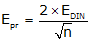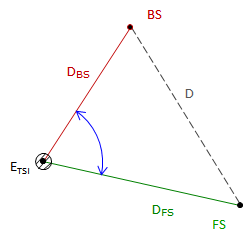## 4. Combined random errors

(Most of the material in this section has been adapted from Chapter 6 of Adjustment and Computations, first edition, by Wolf & Ghilani).

Many surveyors presume the angle display resolution is the angle measuring accuracy of the TSI. For example, if the TSI display can be set to show angles to 1" then the angle measuring accuracy must be 1". This is no different than a measuring a distance with a tape having a smallest division of 0.01 ft; because you can measure to 0.01 ft doesn't mean your measurement is accurate to 0.01 ft. Holding the 0 end of the tape over the mark will be subject to random error as will reading the tape. Propagating individual random errors will affect the error in the final measurement. Instrument resolution is but one of those errors.

Random errors affecting an angle measurement are can be divided into three categories, Figure E-9:Figure E-9 Centering and Sighting Errors

### a. TSI Pointing and Reading error, Epr

This is a characteristic of the instrument itself much like the MSA for electronic distance measurement.

The DIN 18723 standard was developed as a way to express the expected angle uncertainty of a digital instrument. The standard is more a statement of precision rather than accuracy. A later parallel standard, ISO 17123:3, was developed for the same purpose as DIN 18723 but using different calibration criteria. Many instrument manufacturers have adopted the ISO standard in place of the DIN. The instrument manual should show one or the other in the TSI specifications.

The relationship between the DIN 18723 or ISO 17123:3 precision, EDIN, and the expected combined pointing & reading error in a measured angle, Epr, is:Equation E-3

EDIN and Epr are both in seconds of arc.

### b. TSI Centering error, Etsi

The error due to TSI centering is:Equation (E-4) Etsi: Angle error due to TSI centering; sec Ei:TSI centering error; linear units DBS, DFS:Distances to BS and FS targets; linear units. D: Distance between BS and FS targets; linear units

Figure E-10
TSI Centering Error

### c. Target Centering error, EtEquation E-5 Et: Angle error due to target centering; sec EBS, EFS: BS and FS targets centering errors; linear units. DBS, DFS:Distances to BS and FS targets; linear units.
Figure E-11
Target Centering Errors

### d. Combined errors

The three errors are propagated as the Error of a Sum to determine the error in the measured angle:Equation E-6